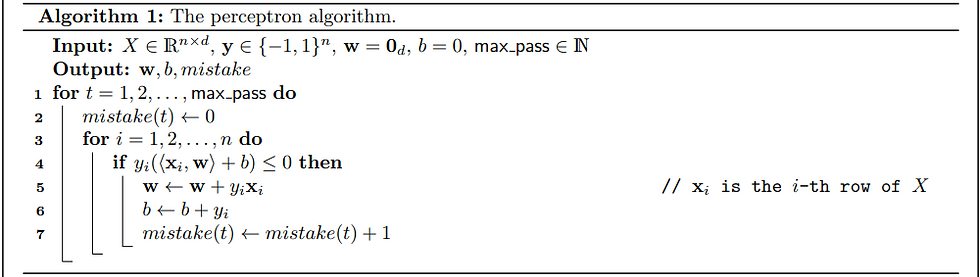top of page
Search

# Theoretical & Mathematical Machine Learning Assignment Help | Sample Paper

Exercise 1: Perceptron Implementation

Convention: All algebraic operations, when applied to a vector or matrix, are understood to be element-wise (unless otherwise stated).Implement the perceptron in Algorithm 1. Your implementation should take input as X = [x1 , . . . , xn^T ]^T ∈ R^n×d , y ∈ {−1, 1}^n, an initialization of the hyperplane parameters w ∈ R^d and b ∈ R, and the maximum number of passes of the training set [suggested max pass = 500]. Run your perceptron algorithm on the spam base dataset (use the version on the course website), and plot the number of mistakes (y-axis) w.r.t. the number of passes (x-axis).

Exercise 2: Regression Implementation

Recall that ridge regression refers to2. (a) Solve the Lasso and Ridge regression problems using the gradient descent algorithm. The following incomplete pseudo-code of the gradient-descent algorithm may be of help. Your training loss should monotonically decrease during iteration; if not try to tune your step size η, e.g. make it smaller.

Test the gradient descent implementation on the Boston housing dataset (to predict the median house price, i.e., y). Use the train and test splits provided on course website. Try λ ∈ {0, 10} and report your training error, training loss, and test error. Plot the training loss over iterations. Compare the running time of the two approaches, which is faster? Overall, which approach do you think is better? Explain why(b) Implement the Ridge regression algorithm using the closed form solution for linear regression. Do not use any library like Scikit Learn that already has linear regression implemented. Feel free to use general libraries for array and matrix operations such as numpy. You may find the function numpy.linalg.solve useful.

(c) Test Ridge regression implementation on the Boston housing dataset (to predict the median house price, i.e., y). Use the train and test splits provided on course website. Try λ ∈ {0, 10} and report your training error, and test error for each. Do you think gradient descent is better than the closed form solution of Ridge regression? Explain why.

Exercise 3: Nearest Neighbour Regression

1. Implement k-nearest neighbour regression, for a dataset of n X, y pairs where X ∈ R^d and y ∈ R. This is similar to k-nearest neighbour classification, but instead of aggregating labels by taking the majority, we average the y values of the k nearest neighbours. Use ℓ2 distance as the distance metric, that is, the distance between points X1 and X2 is ∥X1 − X2∥2. Ensure that your implementation is O(nd) time for all values of k, and argue that this is the case.

2. For the training sets of the d = 1 mystery datasets D and E on the course website, compute a) the (unregularized) least squares linear regression solution and b) the k-nearest neighbour regression solution with each integer k from 1 to 9. For each dataset, on one plot with the x and y axes corresponding to the x and y values, display the least squares solution, the 1-nearest neighbour solution, and the 9-nearest neighbour solution. Be sure to plot these solutions for all points between the minimum and maximum x values, not just for the points in the dataset. On another plot, with k on the x axis and test mean-squared error on the y axis, display the error of the k-NN solution for each value of k. On the same plot, include the test error of the least squares solution as a horizontal line. When does each approach perform better, and why?

3. For the training set of the d = 20 mystery dataset F on the course website, compute a) the (unregularized) least squares linear regression solution and b) the k-nearest neighbour regression solution with each integer k from 1 to 3. Plot, with k on the x axis and test mean-squared error on the y axis, display the error of the k-NN solution for each value of k. On the same plot, include the test error of the least squares solution as a horizontal line. Which approach performs better, and why? Hint: consider inspecting distances between the test points and their k nearest neighbours in the training set. 4. (Extra Credit: 5 pts) For the training set of the d = 20 mystery dataset F on the course website, find the best k for k-nearest neighbours regression using 10-fold cross-validation. Draw a graph that shows the cross validation error as k increases from 1 to 30 in increments of 1. Report the best k and the error of k-nearest neighbours on the test set for this best k.

Hire expert to get help in any mathematical or theoretical based machine learning assignment.